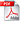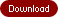How to Solve Large Linear Systems

Using a Stable Cybernetic Approach for Non-Cumulative Computation, Avoiding Underflow and Overflow, with Unconditional and Uniform Convergence

by Aleksa Srdanov and Aleksandra JankovicAuthor website: https://www.researchgate.net/profile/Aleksa_Srdanov
• Number of Pages: 72
• ISBN-10: 1627347380
• ISBN-13: 9781627347389
• Publisher: BrownWalker Press
• Year: 2019
• Category: Mathematics,View First 25 Pages:(free download)

Synopsis

Solving the linear equation system n x n can also be a problem for a computer, even when the number of equations and unknowns is relatively small (a few hundred). All existing methods are burdened by at least one of the following problems: 1) Complexity of computation expressed through the number of operations required to be done to obtaining solution; 2) Unrestricted growth of the size of the intermediate result, which causes overflow and underflow problems; 3) Changing the value of some coefficients in the input system, which causes the instability of the solution; 4) Require certain conditions for convergence, etc.

In this paper an approximate and exact methods for solving a system of linear equations with an arbitrary number of equations and the same number of unknowns is presented. All the mentioned problems can be avoided by the proposed methods.

It is possible to define an algorithm that does not solve the system of equations in the usual mathematical way, but still finds its exact solution in the exact number of steps already defined. The methods consist of simple computations that are not cumulative. At the same time, the number of operations is acceptable even for a relatively large number of equations and unknowns. In addition, the algorithms allows the process to start from an arbitrary initial n-tuple and always leads to the exact solution if it exists.